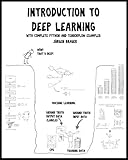# Introduction to Deep Learning: with complete Python and TensorFlow examplesIntroduction to Deep Learning: with complete Python and TensorFlow examples

By 作者: Juergen Brauer
ISBN-10 书号: 1724716417
ISBN-13 书号: 9781724716415
Release Finelybook 出版日期: 2018-08-03
pages 页数: 246

\$58

In Computer Sciences there is currently a gold rush mood due to a new field called “Deep Learning”.
But what is Deep Learning? This book is an introduction to Neural Networks and the most important Deep Learning model – the Convolutional Neural Network model including a description of tricks that can be used to train such models more quickly.
We start with the biological role model: the Neuron. About 86.000.000.000 of these simple processing elements are in your brain! And they all work in parallel! We discuss how to model the operation of a biological neuron with technical neuron models and then consider the first simple single-layer network of technical neurons. We then introduce the Multi-Layer Perceptron (MLP) and the Convolutional Neural Network (CNN) model which uses the MLP at its end. At the end of the book we discuss promising new directions for the field of Deep Learning.
A famous physicist once said: “What I cannot create, I do not understand”. For this, the book is full of examples of how to program all models discussed in Python and TensorFlow – Today, the most important Deep Learning library.

Introduction to Deep Learning with complete Python and TensorFlow examples 9781724716415.pdf

### 觉得文章有用就打赏一下文章作者

#### 支付宝扫一扫打赏#### 微信扫一扫打赏• QQ咨询
• 回顶